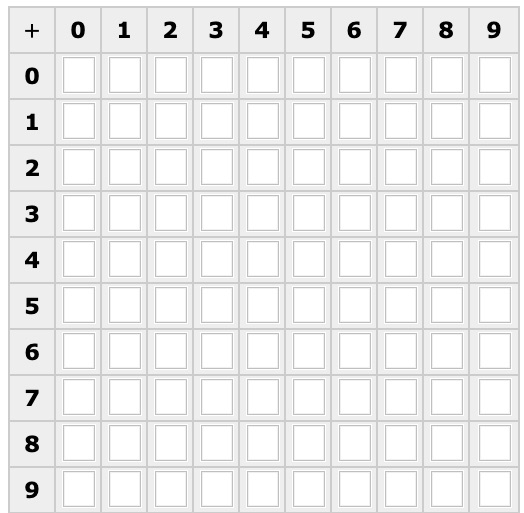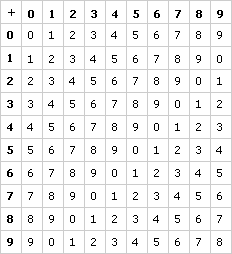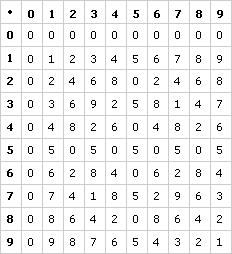# What Is a Number System? Part A: A Simpler Number System (70 minutes)

In This Part: Addition in Units Digit Arithmetic
Stated simply, a number system is a set of objects (often numbers), operations, and the rules governing those operations. One example is our familiar real number system, which uses base ten numbers and such operations as addition and multiplication. Another example is the binary number system, which uses binary addition and multiplication.

Gaining an understanding of the real number system’s elements, operations, and rules is inherently difficult. One important reason for this is that the system has an infinite or unlimited number of elements. Although we use this system every day, we usually don’t think much about it when we use it.

Before we begin to analyze the real number system, we will first examine a finite number system — its elements (which, unlike the real number system, are limited in number), its operations, and the rules that govern it. You will see that this system follows some (but not all) of the same rules as the real number system.

To begin, suppose that when you add or multiply whole numbers, you only need to keep track of the units digit. Only the units digit of the original numbers affects the answer, and you record only the units digit of your answer.

Thus, we can think of this as a system that includes only the digits 0, 1, . . ., 9 and, for now, only the operations of addition and multiplication. Let’s see what patterns emerge as we explore this finite number system.

Problem A1
In units digit arithmetic, 9 + 5 = 4 (as opposed to our regular system, in which 9 + 5 = 14), because we are only interested in the units digit. Fill in the addition table below, using units digit arithmetic.Problem A2
a.
What patterns do you observe in this addition table?
b.
What do you think is responsible for the diagonal patterns you see?Pick two numbers, one from the top horizontal row and the other from the vertical column on the far left, and add them. See where you land on the table. Then consider what happens if you move one up from the original number in the vertical column and one to the right in the horizontal row.Video Segment
In this video segment, Rhonda and Monique discuss patterns they noticed in the finite system. Next, the whole class begins to think about why those patterns occur. Watch this segment after you have completed Problems A1 and A2, and then compare your findings with those of the onscreen participants.

Did you notice any additional patterns? Can you explain why they occur?

You can find this segment on the session video approximately 4 minutes and 16 seconds after the Annenberg Media logo.

In This Part: Inverse, Identity, and Closure

In number systems, it is sometimes useful to find identity and inverse elements. Such elements can tell us more about the behavior of numbers in a particular system. Let’s explore what this means.

The identity element for addition (i.e., the additive identity element) is a number that, when added to any other number in the table, doesn’t change its value.

The inverse element for addition (i.e., the additive inverse element) is a number that, when added to any other number in the table, gives back the identity element.

What do these elements tell us about the finite number system?Problem A3
Find the additive identity element in the addition table you created. Is there one number that works for every other number in your table?

Problem A4
a.  Find the additive inverse element for the number 4 in the table you created.Remember, the additive identity element for this number system is 0.

b. Find the additive inverse for as many of the elements in your set as you can. Does every number in your table have an additive inverse?

Problem A5

a. The commutative law for addition states that a + b = b + a. Does this law hold for the finite number system in your table? Why or why not? Note 1

b. The associative law for addition states that (a + b) + c = a + (b + c). Does this law hold for the finite number system in your table? Why or why not?

Problem A6

a. How could you use the addition table to subtract?Consider subtraction as a way of undoing addition. Think of what number you’d need to add to the second number in the subtraction problem in order to obtain the first number (a – b = x; or b + x = a).

b. Is it possible to subtract any number from any other number using this number system?

A set is said to be closed under a given operation if the result of the operation is always in the set. For example, the integers as we know them are closed under addition, because whenever you add two integers, you get an integer. They are not closed under division because 5 divided by 3 is not in the set — it is not an integer.

Problem A7
Is this finite set closed under addition?
Note that you can further explore units digit arithmetic in Learning Math: Patterns, Functions, and Algebra, Session 9.

In This Part: Multiplication in Units Digit Arithmetic
Problem A8
Fill in the multiplication table below using units digit arithmetic. For example, in our regular system, 6 • 3 = 18. But in units digit arithmetic, we are only interested in the units digit, so in this system, 6 • 3 = 8.Pattern A9
a.
What patterns do you observe among the numbers in your table?
b. Why do you think these patterns occur?

In This Part: Multiplicative Inverse and Identity
Once more, we can look for the identity and inverse elements for multiplication. Remember, we are interested in these elements in order to have a better understanding of the behavior of numbers in a particular number system.

The multiplicative identity element is a number that, when multiplied by any other number in the table, doesn’t change its value.

The multiplicative inverse element is a number that, when multiplied by any other number in the table, gives back the identity element.Problem A10
a.
Find the multiplicative identity element for the multiplication table you created.
b. Is there one number that works for every number in your table?

Problem A11
a. Find the multiplicative inverse element for the number 3 in the multiplication table you created
b. Find the multiplicative inverse for as many of the numbers in your table as you can.
c. Separate the elements into two sets — those with and those without inverses. What similarities and differences can you observe between the two sets?Video Segment
In this video segment, Kristen and Nancy contemplate the inverse and identity elements for the multiplication table in the finite number system. They notice some interesting patterns, which the whole class then discusses. Watch this segment after you’ve completed Problems A8-A11.

Can you think of any other sets that are dense?

You can find this segment on the session video approximately 10 minutes and 9 seconds after the Annenberg Media logo.

### Solutions

Problem A1
Here is the completed table:Problem A2
a.
Answers will vary. Many people will notice that the table is symmetrical; if you look at any two points directly across the main diagonal (from top left to bottom right), you will see that the values are equal. Other patterns include the fact that the same number appears on each diagonal from bottom left to top right (one diagonal has nothing but 9s, for example), and the fact that each number appears in every row and column exactly once.
b. The diagonal pattern exists because these are all numbers that add up to the same value in the real number system. Moving one unit up reduces the sum by 1, and moving one unit to the right increases the sum by 1, so moving along this type of diagonal (up and right, or down and left) does not change the sum of the two numbers.

Problem A3
The number is 0. Zero plus any number in the system equals that number. For example, 7 + 0 and 0 + 7 both equal 7.

Problem A4
a. For the number 4, we are looking for a number x so that 4 + x = 0 in the system. We want a value so that 0 will be the units digit of the sum. If the sum is 10, then 0 will be the units digit. Therefore, x is 6, and 4 + 6 = 0 in the system.

b. Yes, every number in the table has an additive inverse. Here is a table:

NumberInverse00 1 9 2 8 3 7 4 6 5 5 6 4 7 3 8 2 9 1

Note that 0 and 5 are their own inverses.

Problem A5
a.Yes, this law holds. The main reason is that the law is true in real numbers, so it must also be true in this system (which is based directly on real number arithmetic). You can also see this from the table; (a + b) and (b + a) are opposite each other on the main diagonal.
b. Yes, this law holds, since the same law is true in real numbers.

Problem A6
a. Finding the answer to 7 – 3 is the same as finding a number x so that 3 + x = 7 in the system. To find this number, we can look at Row 3 in our table to see all the possible results we can get from 3 + x. In this case, the result in Column 4 gives 7, so 3 + 4 = 7, and 4 (the column value) is the solution to 7 – 3.

As a more complicated example, let’s find 2 – 9. This is the same as finding a number x so that 9 + x = 2 in the system. Looking in the row for 9, we want to find a result of 2. This happens in Column 3, so we know that 9 + 3 = 2 and that 3 is the solution to 2 – 9.

b. Yes, it is possible to subtract any number from any other number in this system. This is true because each number occurs in every row and column exactly once, so we can always find a solution to a + x = b, no matter what numbers a and b are.

Problem A7
Yes, definitely. When we add two numbers in the system, we always get a number in the system. Remember that we are looking at only the units digit of the solution, so 6 + 7 = 3, not 13.

Problem A8
Here is the completed table:Problem A9
a. Answers will vary. For example, one pattern is that the first row and column are all zeros. Another is that the table is symmetrical about its main diagonal. Another is that some (but not all) rows have all 10 numbers.
b. Answers will vary. Some patterns are pretty easy to explain — every number multiplied by 0 is 0, so the row and column for 0 should be nothing but zeros. Some are much more difficult to explain, such as which rows will have all 10 numbers.

Problem A10
a. Let’s start with 3. We want to find a number m so that m • 3 = 3 • m = 3. Looking at the row and column for 3, the only number that works is m = 1.

b. Yes, m = 1 works for every number in the table.

Problem A11
a. We want to find a number f so that 3 • f = 1. Looking at the row for 3, the only number that works is f = 7. So 7 is the multiplicative inverse of 3.

b. The inverse of 1 is 1. The inverse of 3 is 7. The inverse of 7 is 3. The inverse of 9 is 9. These are the only numbers that have inverses in this system.c. Numbers with inverses: 1, 3, 7, 9.
Numbers without inverses: 0, 2, 4, 5, 6, 8.All the numbers with inverses are odd, while every even number has no inverse. The only exception to this pattern is 5; according to the multiplication table, any number multiplied by 5 will have a units digit of 0 or 5, so 1 is never a units digit. More explicitly, the numbers with inverses are relatively prime to 10 (they have no common factors, except 1, with 10). The numbers without inverses are not relatively prime to 10; they have common factors with 10 that are greater than 1.